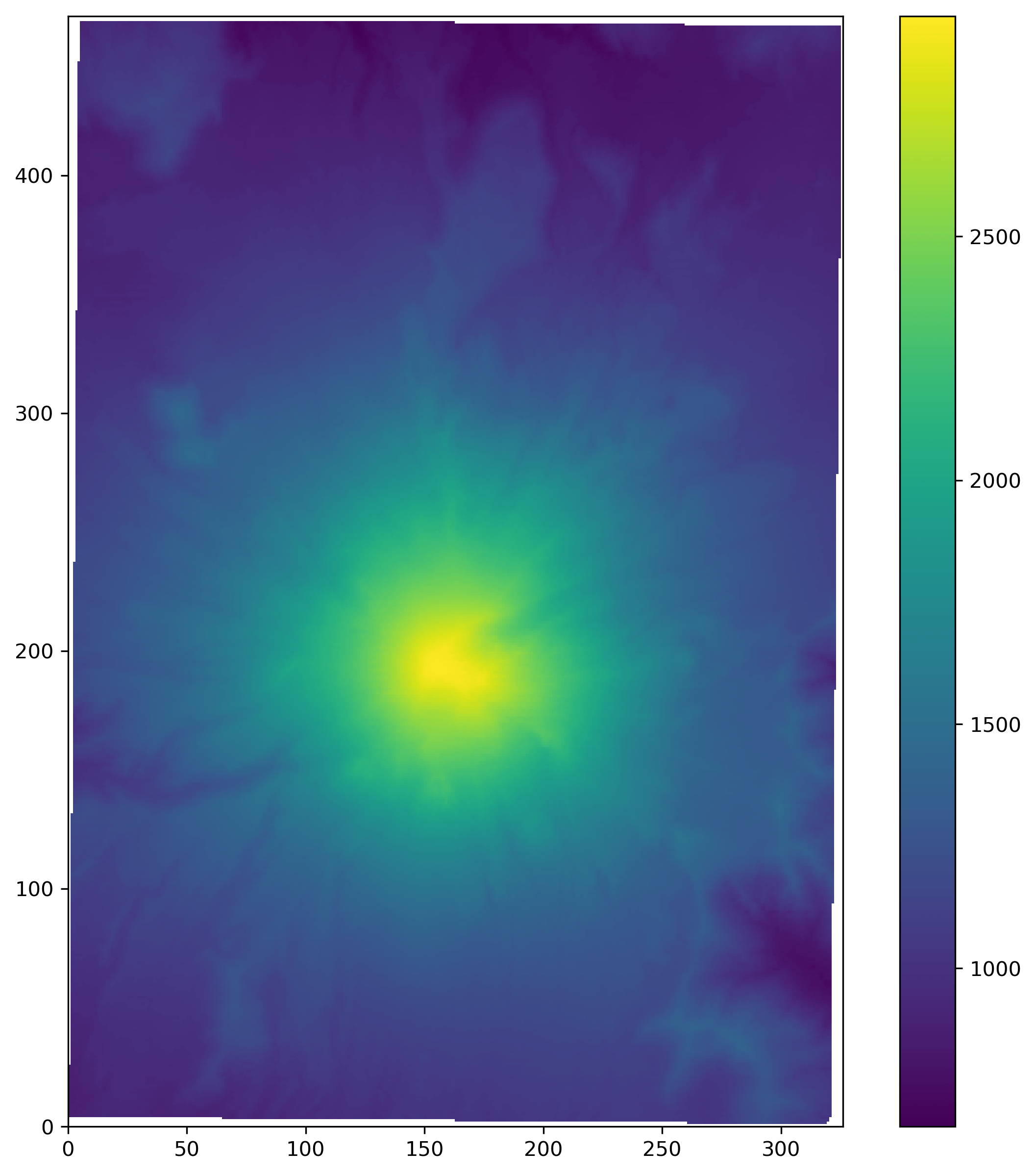# Working with ZMAP+ grid files in Python

I just added to the awesome Geoscience I/O library a notebook showing how to use Python to read in an XYZ grid file in legacy ZMAP+ format as `numpy` array, display it with `matplotlib`, write it to a plain ASCII XYZ grid text file. I will use the DEM data for Mount St. Helens BEFORE the 1980 eruption, taken from Evan Bianco’s excellent notebook, which I converted to ZMAP+ (that part I will demonstrate in a future post / notebook).

You can get the full notebook on GitHub.

ZMAP plus files have a header section that looks something like this (this one comes from the DEM file I am using):

```! -----------------------------------------
! ZMap ASCII grid file
!
! -----------------------------------------
15, 1E+030, , 7, 1
468, 327, 0, 326, 0, 467
0. , 0. , 0.
@
```

where the rows starting with a `!` symbol are comments, and the rows in between the two `@` symbols store the information about the grid. In particular, the first two entries in the third line of this column are `nrows` and `ncols`, the number of rows and columns,  respectively.

The header section is followed by a data section of `ncols` blocks each containing `nrows` elements arranged in rows of 5 (given by the last number of the first row with the `@` symbol.

OK, let’s get to it.

I break the task of reading in two parts: the first part is to gather from the header the information I will be using. I want from the section between the two `@`s:

• the second entry in its second line – the null value
• the first two entries in its third line – the number of rows and columns, as mentioned
• the last four entries in its third line – the xmin, xmax, ymin, ymax, respectively

I am making the assumption that the number of commented rows may not be the same in all ZMAP+ files, hence I abandoned my first approach which was based on using `getline` with specific line numbers. Instead, I used:

• an `if` statement to check for the line beginning with `@` (usually followed by a grid name) but not `@\n`; then grab the next two lines
• another `if` statement to check whether a line starts with `!`
• a counter is increased by one for each line meeting either of the above conditions. This is used later on to skip all lines that do not have data
```filename = '../data/Helens_before_XYZ_ZMAP_PLUS.dat'
count = 0
hdr=[]
with open(filename) as h:
for line in h:
if line.startswith('!'):
count += 1
if line.startswith('@') and not line.startswith('@\n'):
count += 1
hdr.append(next(h)); count += 1
hdr.append(next(h)); count += 1
count += 1```

which gives:

```hdr
>>> ['15, 1E+030, , 7, 1\n', '468, 327, 0, 326, 0, 467\n']```

Next I use the line `hdr = [x.strip('\n') for x in ','.join(hdr).split(',')]` to remove the `newline`, and the next `if` / `else` statement to deal with the null values, if present:

```if hdr ==' ': null=np.nan
else: null = float(hdr)```

Finally, I get the remainder of the information with:

```xmin, xmax, ymin, ymax = [ float(x) for x in hdr[7:11]]
grid_rows, grid_cols = [int(y) for y in hdr[5:7]]```

Which I can check with:

```print(null, xmin, xmax, ymin, ymax, grid_rows, grid_cols)
>>> 1e+30 0.0 326.0 0.0 467.0 468 327
```

That’s it! I think there is room to come back and write something more efficient later on, but for now I am satisfied this is robust enough to handle headers.

The rest, reading in the data, is downhill, with a first loop to skip the 9 header lines, and a second one to read all elements in a single array:

```with open(filename) as f:
[next(f) for _ in range(count)]
for line in f:
grid = (np.asarray([word for line in f for word in line.split()]).astype(float)).reshape(grid_cols, grid_rows).T
grid[grid==null]=np.nan
```

Done!!